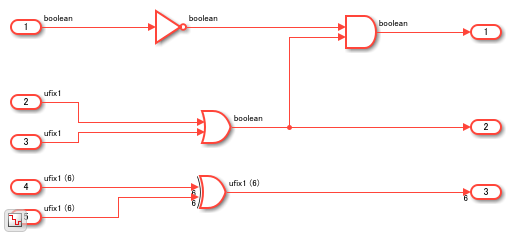## Usage of Blocks in Logic and Bit Operations Library

These guidelines illustrate how to model your design for generating HDL-ready code from blocks in the Logic and Bit Operations Library. The Library contains blocks that perform logical and bitwise operations, bit reduction, and concatenation. Each guideline has a severity level that indicates the level of compliance requirements. To learn more, see HDL Modeling Guidelines Severity Levels.

### Logical and Arithmetic Bit Shift Operations

`2.2.1`

Informative

#### Description

You can use Simulink® blocks to perform bit shifting operations. The blocks can perform logical and arithmetic bit shift. Left logical and arithmetic bit shift produce the same results but right logical shift and arithmetic shift operate differently as illustrated in this table.

Block/Function NameParameter/OperationVerilog Code EquivalentVHDL code equivalentComments
Bit Shift`Shift Left Logical``<<<``sll` (`sll` and `SHIFT_LEFT` are the same in VHDL.This mode is the default mode for the block. The left shift operation does not preserve the sign bit. If the input uses a signed data type and has a positive value, the left shift operation shifts a `0` into the empty bit on the LSB (Least Significant Bit) side.
`Shift Right Logical``>>``srl`This mode does not preserve the sign bit. If the input uses a signed data type and has a positive value, the right shift operation shifts a `0` into the empty bit on the MSB (Most Significant Bit) side.
`Shift Right Arithmetic``>>>``SHIFT_RIGHT`When the input is a signed data type, the sign bit is preserved, and other bits shift to the right.
Shift Arithmetic block `bitshift` functionPositive value/shift right arithmetic`>>>``SHIFT_RIGHT`When the input is a signed data type, the sign bit is preserved, and other bits shift to the right.
Negative value/shift left arithmetic`<<<``sll`This mode does not preserve the sign bit. If the input uses a signed data type and has a positive value, the left shift operation shifts a `0` into the empty bit on the LSB side. This mode does not check underflows and overflows.
`bitsll` functionNone/logical left shift`<<<``sll`This mode does not preserve the sign bit. The generated HDL code is the same as that of the ```Shift Left Logical``` mode of the Bit Shift block.
`bitsrl` functionNone/logical right sift`>>``srl`This mode does not preserve the sign bit. The generated HDL code is the same as that of the ```Shift Right Logical``` mode of the Bit Shift block.
`bitsra` functionNone/arithmetic right shift`>>>``SHIFT_RIGHT`When the input is a signed data type, the sign bit is preserved, and other bits shift to the right. The generated HDL code is the same as that of the ```Shift Right Arithmetic``` mode of the Bit Shift block.

The difference between a logical shift and an arithmetic shift is whether the sign bit is preserved. For signed data types, this bit is the MSB. In a logical right shift, the sign bit is shifted to the right and zero goes into the MSB side. In an arithmetic right shift, the MSB (sign bit) is preserved during the shift operation. For example, this code illustrates the difference between the functions.

```A = fi([], 1, 4, 0, 'bin','1011'); B = bitsrl(A, 2)```
```B = 2 DataTypeMode: Fixed-point: binary point scaling Signedness: Signed WordLength: 4 FractionLength: 0```
`B.bin`
```ans = '0010'```
`C = bitsra(A, 2)`
```C = -2 DataTypeMode: Fixed-point: binary point scaling Signedness: Signed WordLength: 4 FractionLength: 0```
`C.bin`
```ans = '1110' ```

### Usage of Logical Operator, Bitwise Operator, and Bit Reduce Blocks

Guideline ID

`2.2.2`

Severity

Informative

Description

For an example of logical and bitwise operations, open the model `hdlcoder_logical_bitwise_operations.slx`.

```load_system('hdlcoder_logical_bitwise_operations') sim('hdlcoder_logical_bitwise_operations') open_system('hdlcoder_logical_bitwise_operations') ```For single-bit operations that use `Boolean` or `ufix1` data types, use a Logical Operator block. To view the operation as a logical circuit symbol, in the Block Parameters dialog box of the block, specify the Icon shape as `Distinctive`. You can also input vectors that have `Boolean` or `ufix1` data types to the block.

`Boolean` and `ufix1` are different data types. Avoid using both data types within the same model, or using them interchangeably. See Simulink Data Type Considerations.

For an example of using the block, open the `Logical Operations` Subsystem.

```open_system('hdlcoder_logical_bitwise_operations/Logical Operations') ```For bitwise operations on two or more bits that use integer or fixed-point data types, use the Bitwise Operator block. For an example, double-click the `Bitwise Operations` Subsystem.

```open_system('hdlcoder_logical_bitwise_operations/Bitwise Operations') ```To perform a bit-by-bit reduction operation on a vector that uses `Boolean` or `ufix1` and return a 1-bit value, use the Bit Reduce block. For an example, double-click the `Reduction Operations` Subsystem.

```open_system('hdlcoder_logical_bitwise_operations/Reduction Operations') ```The MATLAB Function block inside the Subsystem converts the 8-bit vector to a vector of `8` 1-bit `ufix1` elements.

```open_system('hdlcoder_logical_bitwise_operations/Reduction Operations/convert bin2vec') ```

### Use Boolean Output for Compare to Constant and Relational Operator Blocks

`2.2.3`

#### Severity

Strongly Recommended

#### Description

For Compare To Constant, Compare To Zero, and Relational Operator blocks, you can specify `uint8` or `boolean` as the Output data type. To generate efficient HDL code for models that contain these blocks, specify `boolean` as the Output data type, because the HDL code has to connect only the LSB.

For a Relational Operator block, make sure that both inputs are of the same data type. Using different data types for the inputs can result in unintended truncation of bits such as the sign bit, which can lead to simulation mismatches after HDL code generation.

To verify whether Relational Operator blocks in your model use the same input data type, and use `boolean` as the output data type, run the HDL model check Check for Relational Operator block usage.